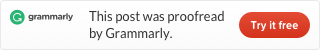Categories

# LeetCode 75 Sort Colors [sort] Java

Source URL: https://leetcode.com/problems/sort-colors/

Given an array with n objects colored red, white or blue, sort them in-place so that objects of the same color are adjacent, with the colors in the order red, white and blue.

Here, we will use the integers 0, 1, and 2 to represent the color red, white, and blue respectively.

Note: You are not suppose to use the library’s sort function for this problem.

Example:

```Input: [2,0,2,1,1,0]
Output: [0,0,1,1,2,2]```

• A rather straight forward solution is a two-pass algorithm using counting sort.
First, iterate the array counting number of 0’s, 1’s, and 2’s, then overwrite array with total number of 0’s, then 1’s and followed by 2’s.
• Could you come up with a one-pass algorithm using only constant space?

This problem is easy. Because whatever how much input, we only got three colors. We can count the number of each color, then loop out of it. This is called the counting sort. Only need one pass for counting, and one pass for output.

My code is :

Running time is 0ms faster than 100% Java submissions.